Question

# Using a pH meter, the pH of a lemon was found to be 2.24. Determine the...

Using a pH meter, the pH of a lemon was found to be 2.24. Determine the [H+]. Titration of a 1.0 ml sample of the above juice to a pH of approximately 7 required 02.34 ml of 0.10M NaOH. Determine the total [H+] in the juice Explain why the above two numbers are not the same.

We know,

pH= -log(H+) …………………………………….1

Given,

pH=2.24

Therefore from equation 1,

2.24= -log(H+)

Or, log (H+) = -2.24

Or, [H+] = 10-2.24

[H+] = 0.00575

Now in the second case,

V1 = 1 ml          V2 =2.34 ml                        (S= concentration of solution)

S1 =? (M)          S2 =0.10 (M)

Now the required formulae,

V1S1 = V2S2

Or, 1 x S1 = 2.34 x 0.10 (putting all the values)

Or, S1 =0.234 (M)

Citric acid is present in lemon. Citric acid has 3 ionizable COOH groups. In presence of NaOH (strong base), it completely ionizes and produces 4 protons which react with NaOH and produces water.

C(OH)(COOH)3 = C(O-1)(COO)33- + 4 H+

Therefore, [H+] = 4 x 0.234

= 0.936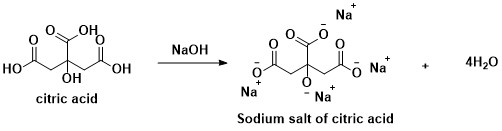The two values are different is due to in presence of strong base (NaOH), OH protons also ionizes and produces protons.

#### Earn Coins

Coins can be redeemed for fabulous gifts.

Similar Homework Help Questions
• ### Vinigar Analysis; General Chemistry

A. Assuming the density of a 5% acetic acid is 1.0 g/mL,determine the volume of the acetic acid solution necessary tonutralize 25.0 mL of 0.10 M NaOH.B. A 31.43 mL colume of 0.108 M NaOH is required to reach thephenolphthlein endpoint in the titration of a 4.441 g sample ofvinegar. Caculate the percent of aceticacid in vinegar.C. Lemon juice has a pH of about 2.5. Assuming that theacidity of a lemon juice is due soley to citric acid, that citricacid...

• ### O solution. If lemon juice sample was neutralized by itration with NaOH son NaOH for neutralization,...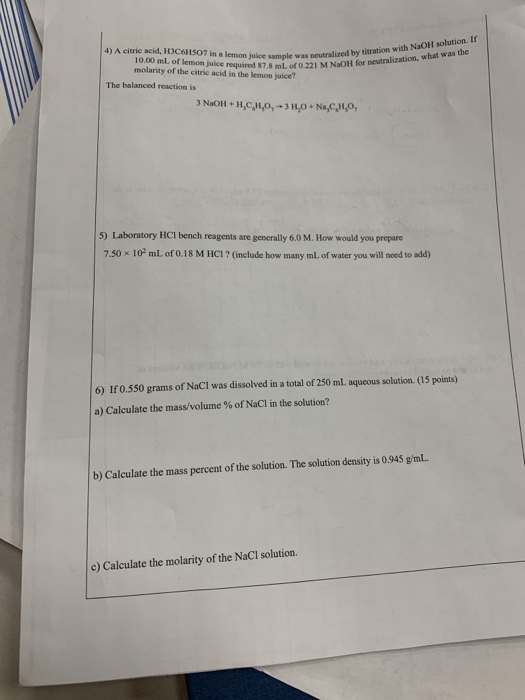O solution. If lemon juice sample was neutralized by itration with NaOH son NaOH for neutralization, what was the 4) A citric acid, HIC6H5O7 in a lemon juice sample was neutral 10.00 mL of lemon juice required 7. ml. 0.221 M N O for cura molarity of the citric acid in the lemon juice? The balanced reaction is 3 NaOH + A,C,H,0,-3 H0 + NaCl,0, 5) Laboratory HCl bench reagents are generally 6,0 M. How would you prepare 7,50 x...

• ### please solve the calculation page DATA SHEET: PH, BUFFERS AND K, Part 1: The Titration of...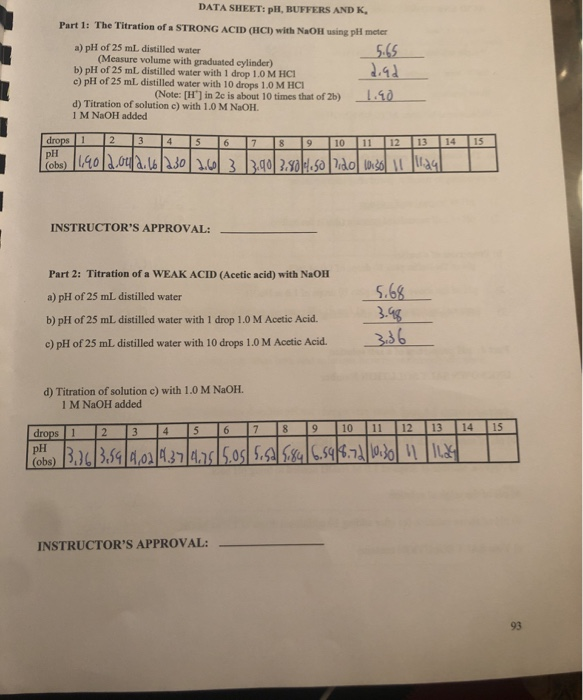please solve the calculation page DATA SHEET: PH, BUFFERS AND K, Part 1: The Titration of a STRONG ACID (HCI) with NaOH using pH meter a) pH of 25 mL distilled water 5.65 (Measure volume with graduated cylinder) b) pH of 25 mL distilled water with 1 drop 1.0 M HCI 2.92 c) pH of 25 mL distilled water with 10 drops 1.0 M HCI (Note: (H') in 2c is about 10 times that of 2b) 1.10 d) Titration of...

• ### A vinegar titration was completed using a pH meter as an indicator of the changing pH....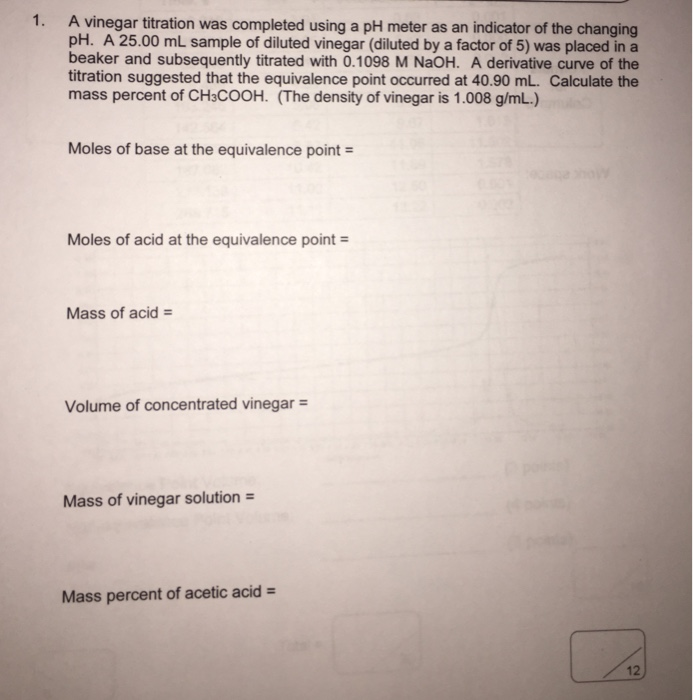A vinegar titration was completed using a pH meter as an indicator of the changing pH. A 25.00 mL sample of diluted vinegar (diluted by a factor of 5) was placed in a beaker and subsequently titrated with 0.1098 M NaOH. A derivative curve of the titration suggested that the equivalence point occurred at 40.90 mL. Calculate the mass percent of CH_3COOH. (The density of vinegar is 1.008 g/mL.) Moles of base at the equivalence point = Moles of acid...

• ### 2) Titration of acetic acid using indicator and pH meter Trial 1 oo Mass of empty...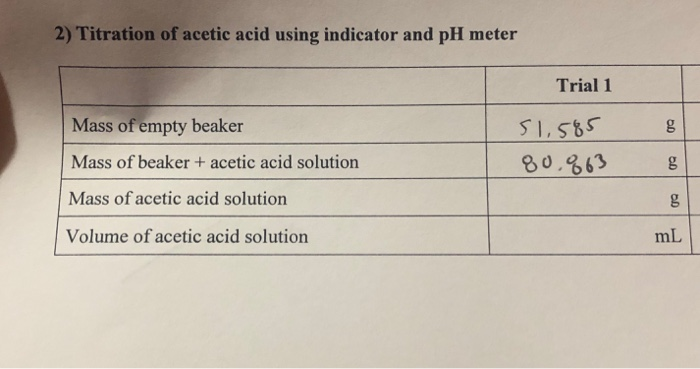2) Titration of acetic acid using indicator and pH meter Trial 1 oo Mass of empty beaker Mass of beaker + acetic acid solution 51.585 80,863 oo Mass of acetic acid solution oo Volume of acetic acid solution mL Record your titration data in the table below. Measure _pH 9.5 54 Vol. of NaOH Vol. of NaOH Measured Vol. of NaOH 1 Vol. of NaOH in buret (mL) added (mL) in buret (mL) added (mL) pH 0.00 248 5.24 10.0...

• ### Need help with this prelab please Experiment 10 Prelaboratory Assignment Vinegar Analysis Lolo Sec. Name...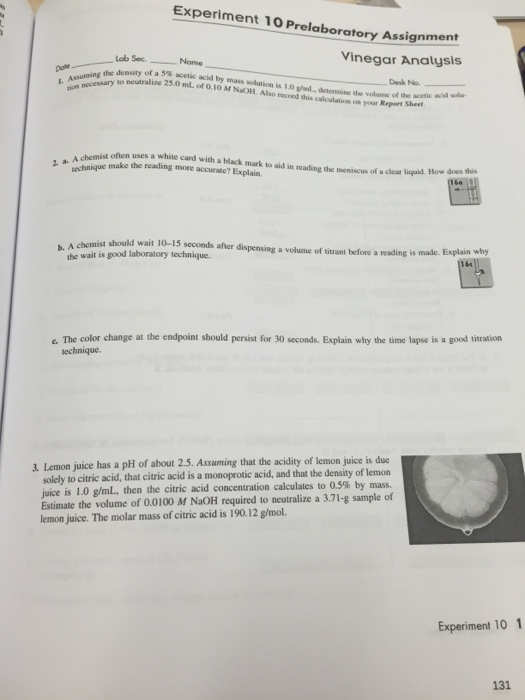Need help with this prelab please Experiment 10 Prelaboratory Assignment Vinegar Analysis Lolo Sec. Name I. Asunning the to of a 59. acetic acid by mass sclution is 10 Desk No. 25.0 ml. of density 0.10 M NaoH Also record desemnine volume of aceic acid" dhis calculation your Report Sheet A chemist often uses a white card with a black mark to aid in reading the meniscus of a technique make the reading more clear liquid. How does this b....

• ### Equivalence Point for Titration #1: 24.96 mL Equivalence Point for Titration #2: 25.40 mL Equivalence Point...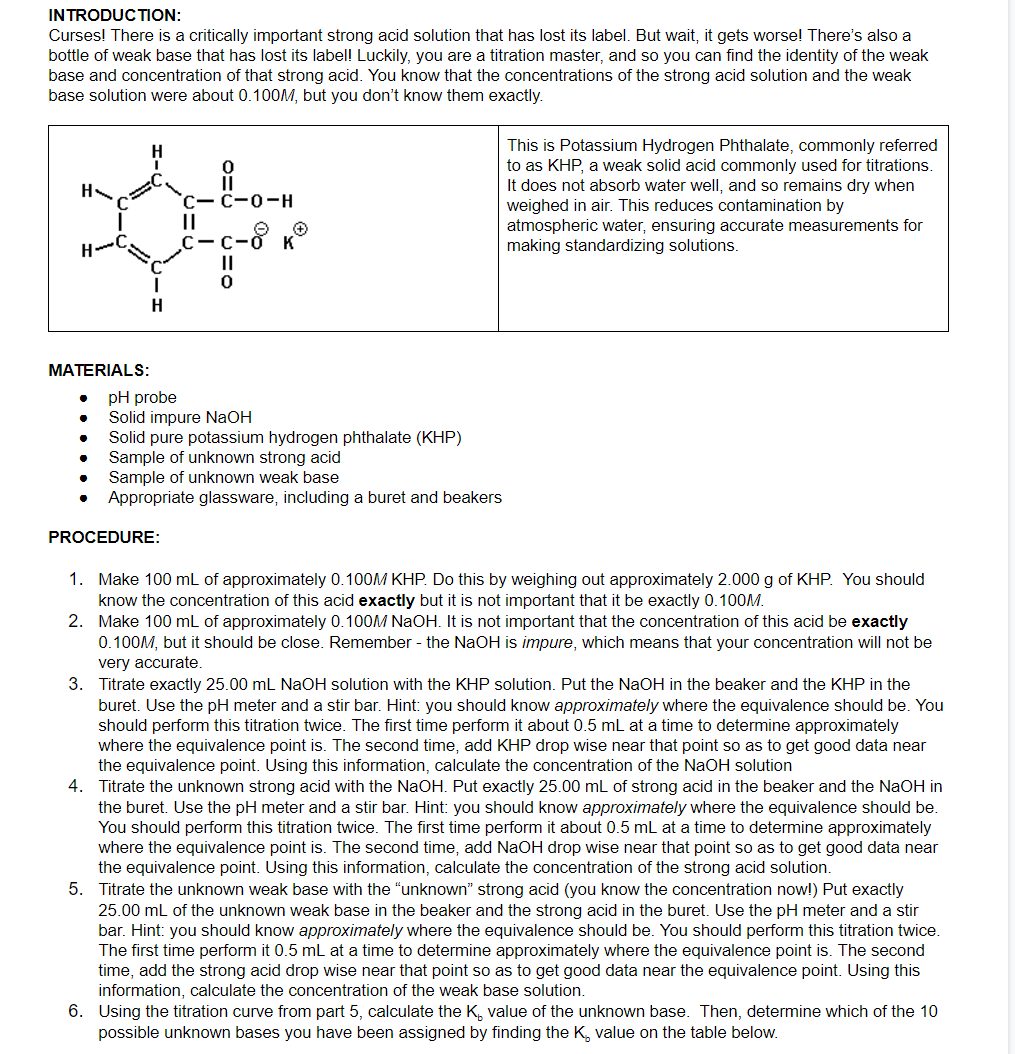Equivalence Point for Titration #1: 24.96 mL Equivalence Point for Titration #2: 25.40 mL Equivalence Point for Titration #3: 25.20 mL Midpoint pH for Titration #3: 9.80 QUESTIONS: 4) Set up the calculation required to determine the concentration of the NaOH solution via titration of a given amount of KHP. Include all numbers except the given mass of KHP. 5) Set up the calculation required to determine the concentration of the unknown strong acid via titration with a known volume...

• ### (b) Another HCl solution is labeled -0.1 M. What do we know about the pH of...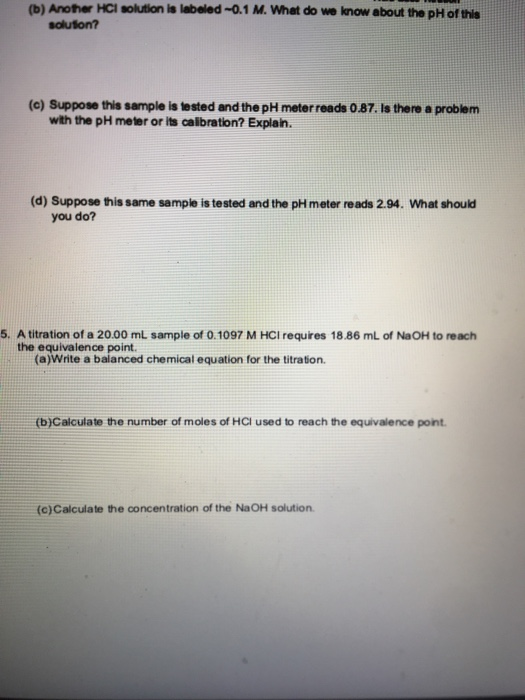(b) Another HCl solution is labeled -0.1 M. What do we know about the pH of this solution? (C) Suppose this sample is tested and the pH meter reads 0.87. Is there a problem with the pH meter or its calibration? Explain. (d) Suppose this same sample is tested and the pH meter reads 2.94. What should you do? 5. A titration of a 20.00 mL sample of 0.1097 M HCl requires 18.86 mL of NaOH to reach the equivalence...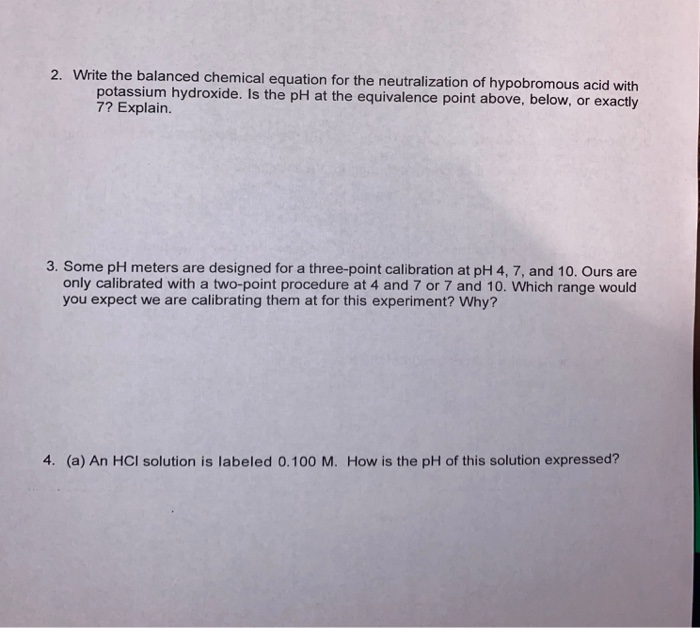please help please help!! 2. Write the balanced chemical equation for the neutralization of hypobromous acid with potassium hydroxide. Is the pH at the equivalence point above, below, or exactly 7? Explain. 3. Some pH meters are designed for a three-point calibration at pH 4, 7, and 10. Ours are only calibrated with a two-point procedure at 4 and 7 or 7 and 10. Which range would you expect we are calibrating them at for this experiment? Why? 4. (a)...

• ### 1) NaOH(aq) + HCl(aq) - NaCl(aq) + H2O(1) To determine the concentration of a NaOH(aq) solution,...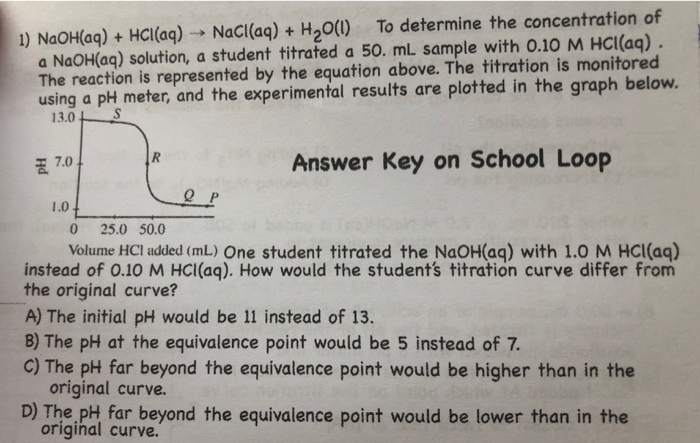1) NaOH(aq) + HCl(aq) - NaCl(aq) + H2O(1) To determine the concentration of a NaOH(aq) solution, a student titrated a 50. mL sample with 0.10 M HCl(aq). The reaction is represented by the equation above. The titration is monitored using a pH meter, and the experimental results are plotted in the graph below. 13. 0S 7.04 Answer Key on School Loop @ P 1.07. 0 25.0 50.0 Volume HCI added (mL) One student titrated the NaOH(aq) with 1.0 M HCl(aq)...